Next: SUPERSTRING PHENOMENOLOGY BEFORE 1995 Up: SUPERSTRING PHENOMENOLOGY AND THE Previous: SUPERSTRING PHENOMENOLOGY AND THE

# Introduction

Quantum field theory (QFT) is the fundamental stone on which high energy physics is based . This theory was developed in order to make consistent the general principles of special relativity and quantum mechanics, both fundamental to the study of elementary particles. Given the generality of QFT, there are very few general consequences we can extract from it. We can only mention: the existence of antiparticles, the running of coupling constants, the relation between spin and statistics and the CPT theorem.

To obtain more concrete information from QFT we need to consider specific models. For this we have a large degree of arbitrariness. We are free to choose the dimension of spacetime, the spin of the particles, the corresponding gauge group, of arbitrary rank, the number of different matter' fields of spin smaller than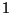and their corresponding representation under the gauge group. Finally we are free to choose the couplings among those fields, renormalizable or not, including the potential for the scalar fields, gauge and Yukawa couplings, etc.

Given that degree of degeneracy, we need to use some experimental input in order to choose an appropriate QFT that could describe our world at least up to a given scale. Such a particular example is the current standard model of particle physics based on the gauge symmetry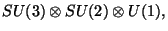with three families of quarks and leptons . This model describes fundamental physics up to a scale of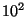GeV where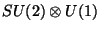is broken to electromagnetic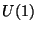. We want to emphasize that this is only one in an infinite number of QFTs and there is no reason other than experimental success to select this model.

Nevertheless, it is widely believed that the standard model is only an effective QFT that has to be generalized to a more fundamental theory. The main reasons for this belief are:

(i) Gravitation is not described at the quantum level. This is probably the most important problem of theoretical high energy physics.

(ii) The gauge hierarchy problem which, roughly speaking, refers to the fact that theGeV scale of symmmetry breaking is not stable under radiative corrections. In the presence of gravity it reduces to the question of why this scale is so small compared with the Planck scale of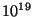Gev.

(iii) There are also the why' problems (Why do we live in 4D?, Why is the gauge group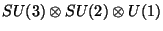?, Why are there three families?, Why do the couplings and masses of the matter fields take the particular values found by experiments? Why is the cosmological constant essentially zero? etc.).

Extensions of the standard model in terms of QFTs are many, and they partially address some of the problems mentioned above. For instance, supersymmetric field theories represent the best candidates to solve the hierarchy problem because the existence of a fermion-boson symmetry can stabilize theGeV scale. There are also grand unified theories (GUTs), which unify the gauge couplings by imposing a simple gauge symmetry such as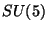, broken to the standard model at higher energies, and also the Kaluza-Klein theories where it is assumed that the world is actually higher-dimensional and the origin of gauge symmetries may be the extra small dimensions of spacetime. All of these extensions of the standard model are just different choices of QFTs and do not address the main problem of theoretical high energy physics, namely the quantization of gravity.

String theory is the only candidate for a fundamental theory of nature, encompassing all the known particles and interactions. In particular, it is the candidate theory for a consistent treatment of gravity in the quantum domain. String theory is not just another extension of the standard model in terms of a QFT, it is a generalization of the QFT itself. Roughly speaking the idea in string theory is to replace the point-like particles of QFT by one-dimensional extended objects, strings, which could be open or closed. Consistency requirements are very strict in string theory, selecting only five supersymmetric theories in 10D, namely: type I with gauge symmetry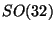, closed type II (A and B depending on orientation properties) and closed heterotic theories with gauge symmetriesor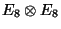. Similar to QFT, string theory has very few general predictions': There is an infinite tower of massive states corresponding to the oscillation modes of the string after quantization, the masses are quantized in terms of a fundamental scale which is identified with the Planck scale. On each of the five different theories there is always a massless particle of spin 2, the graviton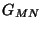, therefore strings imply the existence of gravity. Type I and heterotic have also massless particles of spin,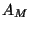, implying the existence of gauge symmetries and are therefore candidates to be the fundamental theory of nature'. The spectrum also includes a singlet massless scalar, the dilaton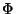and antisymmetric tensors of different ranks depending on the string.

The effective theories describing the massless particles (the masses of observable particles are expected to arise from the ordinary Higgs effect at lower energies) are ordinary QFTs. We can see that string theories then lead to very restricted QFTs, with well defined symmetries and matter content. On the other hand each string theory has many (thousands or billions of) vacua. This allows us to construct string models in any number of dimensions less than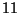, including quasi-realistic models in 4D, which are very similar to the standard model, a very encouraging result. However, it also increases the level of arbitrariness, restricting the predictive power of the theory although, of course, the arbitrariness is still much less than in pure QFT's.

The natural way to construct 4D string models is to start with a 10D theory and use the Kaluza-Klein idea of compactifying six dimensions in small spaces. Most of the variety of different vacua comes from the freedom to choose among these 6D spaces, then 4D string models are sometimes called superstring compactifications (SSC). We can also substitute the 6D space by some 2D conformal field theory (CFT) with specific properties as we will see in the next section.

4D string models are up to now the only candidates for a fundamental theory of nature. Their consistency also requires supersymmetry, which is a welcome property in view of the favourite solution to the hierarchy problem. Strings also improve the `why' problem by changing many of them into a single dynamical question: Why do we live on this particular string vacuum or SSC? This situation is again much better than QFTs, but it is not completely satisfactory. We are left with at least two unsolved problems:

(i) How is supersymmetry broken, in order to recover the (non-supersymmetric) standard model at low energies?
(ii) How do we lift the vacuum degeneracy and select one single SSC describing our low energy world?
Fortunately, string theory is not yet completely understood, and this is not the final status of the theory. String theory is only understood at the perturbative level and non-perturbative questions, such as the tunnelling effect or possible comparison of different vacua, cannot be approached at the moment. A non-perturbative formulation of the theory is expected to give an answer to the why question above, it is also expected to provide the mechanism for the breakdown of supersymmetry at the electroweak scale, hopefully maintaining almost vanishing cosmological constant.

In these lectures I will review the field of string phenomenology. I naturally separate the status of the field before and after 1995. In the first part I will briefly mention the different attempts to construct a quasi-realistic 4D string model, including the obstacles that have been found so far to obtain a realistic model. Next I will discuss what is known about 4D effective actions including the tree-level Yukawa couplings as well as the one-loop corrections to the gauge couplings and nonrenormalization theorems. In chapter 4, I will present some vacuum independent general results of 4D strings. This is the closest we can get to real predictions of 4D string theories so far. Finally I will discuss briefly the problem of supersymmetry breaking and the possible use of duality symmetries in approaching this and other string problems. In the second part of the lectures I mention briefly the progress made during the past 5 years after the ideas of M-theory, D-branes and the Brane-World scenario were introduced.

Since this subject is so vast, I have to restrict to a very superficial discussion; general introductions to string theory and CFT can be found in . There are also two collections of some of the more relevant papers on the subject of 4D strings. Ref. , includes the string model building techniques known before 1989, whereas ref., contains some of the earlier phenomenological discussions of string theory. A number of review articles touching some of the topics of these lectures are provided inNext: SUPERSTRING PHENOMENOLOGY BEFORE 1995 Up: SUPERSTRING PHENOMENOLOGY AND THE Previous: SUPERSTRING PHENOMENOLOGY AND THE
root 2001-01-22